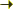Bilbao Crystallographic ServerSupergroupsThe procedure Help

## SUPERGROUPS a computer program for determination of supergroups of space groups.

S. Ivantchev, E. Kroumova, G. Madariaga, J. M. Perez-Mato, M. Aroyo, J. Igartua
Departamentos de Física de la Materia Condensada y Física Aplicada II, Universidad del País Vasco, Apdo 644, 48080 Bilbao, Spain

#### The crystallographic problem

The problem of the determination of the supergroups of a given space group is of rather general interest. It is useful for search of overlooked symmetries in crystal structure determination, or in the analysis of successive phase transitions, where the introduction of a hypothetical (supergroup) parent phase can be important. Another important application is related with the detection of pseudosymmetries in crystal structures as a method of predicting structural phase transitions at higher temperatures (Igartua, Aroyo, Perez-Mato, 1996). There are few papers treating the supergroups of space groups in some detail (Koch, 1984). The existing listings of minimal supergroups of space groups are not complete as they provide only a list of those space groups G which contain a space group H as a maximal non-isomorphic subgroup (International Tables for Crystallography, vol. A, 1992 (ITA)). This information is in general not sufficient because it does not include all the possible supergroups of H isomorphic to G. The presented program determines all possible supergroups Gi of H which belong to the space group type of G.

#### Method of solution

SUPERGROUPS determines all possible supergroups Gi systematically inverting the data on maximal subgroups of space groups (International Tables for Crystallography, vol. A1 (ITA1)). This data has been prepared in CIF-like format in computer-readable form. Following the procedure based in normalizers of space groups (Koch 1984, Wondratschek 1996) the program calculates all supergroups Gi > H, Gi ~ G.

#### Derivation of supergroups

Supergroup: S; Subgroup: G: G <S

The decomposition of S with respect to G:

S = G + q2G + q3G + ... + qnG

Supergroups S(i) of G, isomorphic to S:

S(i) = ai-1 S ai, S(i) ~ S

Decomposition of S(i) with respect to G:

S(i) = ai-1 G ai + ai-1 q2 G ai + ai-1 q3 G ai + ... + ai-1 qn G ai =
= Gi + q2i Gi + q3i Gi + ... + qni Gi, qkj = aj-1 qk aj

S(i) decomposition with respect to G:

S(i) = G + qi,2 G + qi,3 G + ... + qi,n G

==> TWO sets of supergroups S(i) of G:

• Supergroups conjugated under N(G) (the normalizer of G)
S(i) = ai-1 S ai, ai in N(G)

• Supergroups not conjugated under N(G)
S(i) = ai-1 S ai, ai not in N(G)

[SUPERGROUPS]